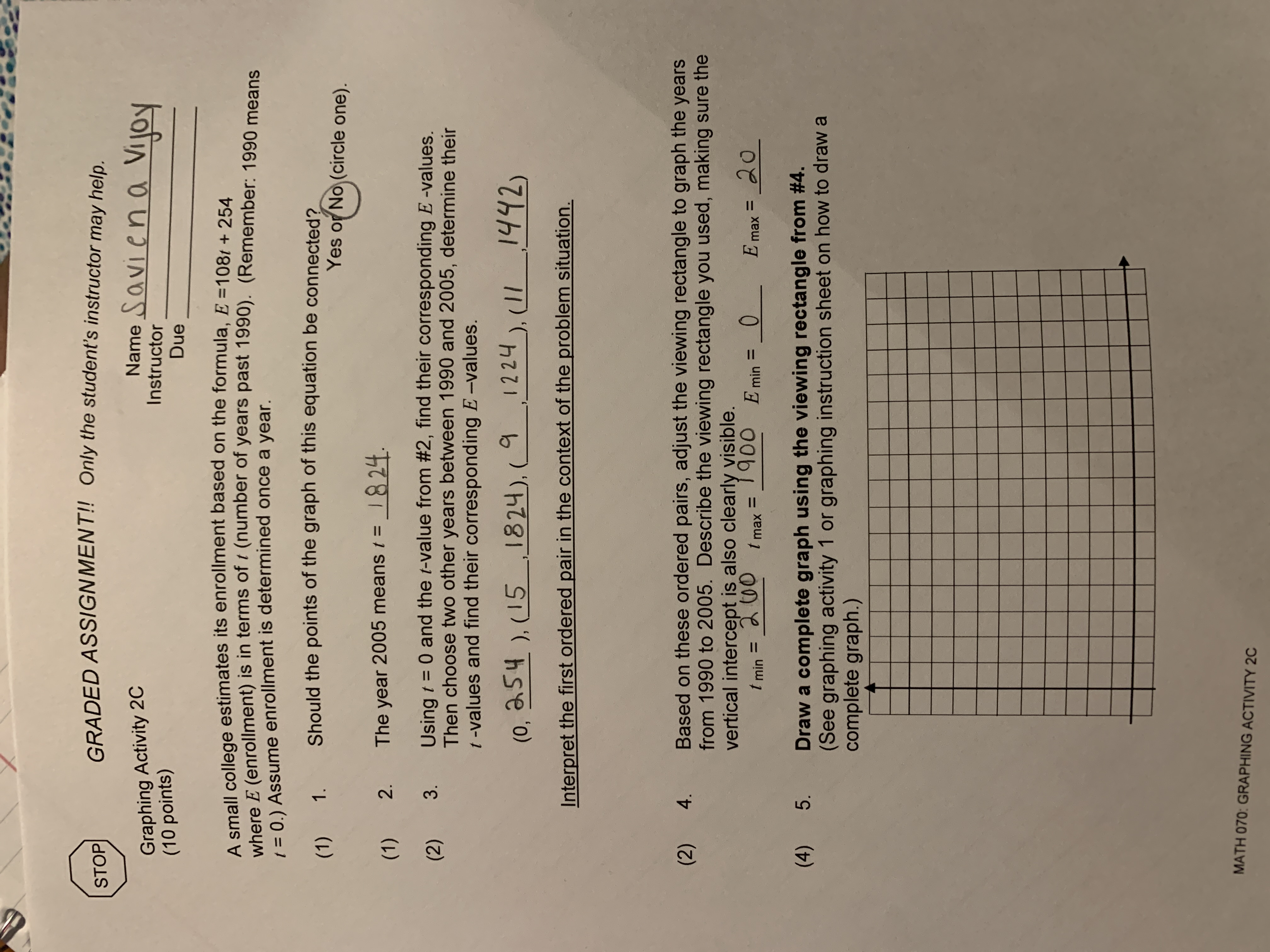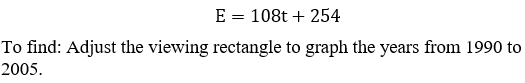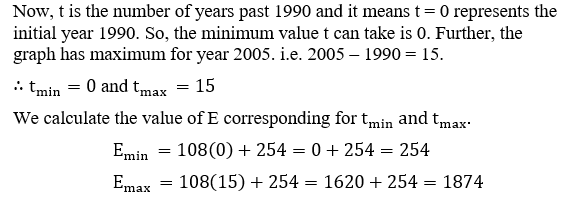# GRADED ASSIGNMENT!! Only the student's instructor may help.STOPName Savien a VijoyGraphing Activity 2C(10 points)InstructorDueA small college estimates its enrollment based on the formula, E =108/ + 254where E (enrollment) is in terms of i (number of years past 1990). (Remember: 1990 means( = 0.) Assume enrollment is determined once a year.Should the points of the graph of this equation be connected?(1) 1.Yes or No (circle one).2.The year 2005 means t =1824.(1)Using t = 0 and the t-value from #2, find their corresponding E -values.Then choose two other years between 1990 and 2005, determine their1 -values and find their corresponding E-values.(2)(0, a54 ), (151824), (_91224), (II_1442)Interpret the first ordered pair in the context of the problem situation.Based on these ordered pairs, adjust the viewing rectangle to graph the yearsfrom 1990 to 2005. Describe the viewing rectangle you used, making sure thevertical intercept is also clearly visible.(2) 4.ang00 Emin202c00 tmaxE max =%3Dt min =%3DDraw a complete graph using the viewing rectangle from #4.(See graphing activity 1 or graphing instruction sheet on how to draw acomplete graph.)(4) 5.MATH 070: GRAPHING ACTIVITY 2C3.

Question
156 views

Number four on the worksheet (image attached) not sure what to set the mins and maxs to.help_outlineImage TranscriptioncloseGRADED ASSIGNMENT!! Only the student's instructor may help. STOP Name Savien a Vijoy Graphing Activity 2C (10 points) Instructor Due A small college estimates its enrollment based on the formula, E =108/ + 254 where E (enrollment) is in terms of i (number of years past 1990). (Remember: 1990 means ( = 0.) Assume enrollment is determined once a year. Should the points of the graph of this equation be connected? (1) 1. Yes or No (circle one). 2. The year 2005 means t = 1824. (1) Using t = 0 and the t-value from #2, find their corresponding E -values. Then choose two other years between 1990 and 2005, determine their 1 -values and find their corresponding E-values. (2) (0, a54 ), (151824), (_9 1224 ), (II_1442) Interpret the first ordered pair in the context of the problem situation. Based on these ordered pairs, adjust the viewing rectangle to graph the years from 1990 to 2005. Describe the viewing rectangle you used, making sure the vertical intercept is also clearly visible. (2) 4. ang00 Emin 20 2c00 tmax E max = %3D t min = %3D Draw a complete graph using the viewing rectangle from #4. (See graphing activity 1 or graphing instruction sheet on how to draw a complete graph.) (4) 5. MATH 070: GRAPHING ACTIVITY 2C 3. fullscreen
check_circle

star
star
star
star
star
1 Rating
Given that a small college estimates its enrollment as below:Calculate the min and max for t and E...

### Want to see the full answer?

See Solution

#### Want to see this answer and more?

Solutions are written by subject experts who are available 24/7. Questions are typically answered within 1 hour.*

See Solution
*Response times may vary by subject and question.
Tagged in